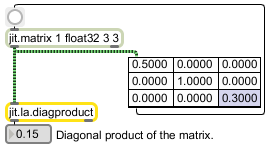# jit.la.diagproduct

Calculate the product across the main diagonal

## Description

The jit.la.diagproduct object calculates the product across the main diagonal of a given input matrix, and send the result out the leftmost outlet. The input matrix must have typefloat32 or float64, and may have planecount 1 or 2. If the input matrix has a planecount of 2, it is assumed that the data is from the set of complex numbers, and the output product is a list of 2 floating point values. The first element is the real component and the second element is the imaginary component.

## Matrix Operator

matrix inputs:1, matrix outputs:0

## Attributes

Name Type g/s Description

## Examples